NEET  >  Law of Inertia & First Law of Motion

# Law of Inertia & First Law of Motion Notes | Study Physics Class 11 - NEET

## Document Description: Law of Inertia & First Law of Motion for NEET 2022 is part of Physics Class 11 preparation. The notes and questions for Law of Inertia & First Law of Motion have been prepared according to the NEET exam syllabus. Information about Law of Inertia & First Law of Motion covers topics like What is the Law of Inertia?, Newton's Laws of Motion - First Law, Frequently Asked Questions – FAQs and Law of Inertia & First Law of Motion Example, for NEET 2022 Exam. Find important definitions, questions, notes, meanings, examples, exercises and tests below for Law of Inertia & First Law of Motion.

Introduction of Law of Inertia & First Law of Motion in English is available as part of our Physics Class 11 for NEET & Law of Inertia & First Law of Motion in Hindi for Physics Class 11 course. Download more important topics related with notes, lectures and mock test series for NEET Exam by signing up for free. NEET: Law of Inertia & First Law of Motion Notes | Study Physics Class 11 - NEET
 Table of contents What is the Law of Inertia? Newton's Laws of Motion - First Law Frequently Asked Questions – FAQs
 1 Crore+ students have signed up on EduRev. Have you?

In the world of Physics, Sir Isaac Newton is the man who pioneered classical physics with his laws of motion. In these laws, the first law is also known as the Law of Inertia. Law of inertia is the most important and renowned one. In this piece of article, let us discuss the first law of inertia in detail. Before discussing the law of inertia, let us know the Inertia Definition. Inertia is defined as a property of matter by which it remains at the state of rest or in uniform motion in the same straight line unless acted upon by some external force.

What is the Law of Inertia?

Law of inertia,  also known as Newton’s first law of motion states that

An object will continue to be in the state of rest or in a state of motion unless an external force acts on it.

We have read about the Aristotle fallacy, as per which an external force is always required to keep a body in motion. This was proved wrong when the concept of inertia came into the picture. With the following two experiments, Galileo established the concept of inertia.

Galileo’s Free Fall Experiment

The most accepted theory of motion in Western philosophy, prior to the Renaissance, was the Aristotelian theory which stated that “In the absence of external power, all objects would come to rest that moving objects only continue to move so long as there is a power inducing them to do so. ” Despite its general acceptance, the Aristotelian theory was discredited by several notable philosophers. Later, Galileo refined the theory of inertia.

How did Galileo Explain Inertia?

Galileo hypothesized that a falling object gains an equal amount of velocity in equal intervals of time. This also means that the speed increases at a constant rate as it falls. But, there was a problem in testing this hypothesis: it was impossible for Galileo to observe the object’s free-falling motion and at the time, technology was unable to record such high speeds. As a result, Galileo attempted to decelerate its motion by replacing the falling object with a ball rolling down an inclined plane. Since free-falling is basically equivalent to a completely vertical ramp, he assumed that a ball rolling down a ramp would speed up in the exact same way as a falling ball would.
Using a water clock, Galileo measured the time it took for the rolling ball to reach a known distance down the inclined plane. After several trials, it was observed that the time it took for the ball to roll the entire length of the ramp was equal to double the amount of time it took for the same ball to only roll a quarter of the distance. In short, if you were to double the amount of distance the ball traveled, it would travel four times as far. Through this experiment, Galileo concluded that

If an object is released from rest and gains speed at a steady rate (as it would in free-fall or when rolling down an inclined plane), then the total distance, s, traveled by the object is proportional to the time squared needed for that travel.

Mathematically, this is expressed as [latex] s α t2 [/latex]

Experiment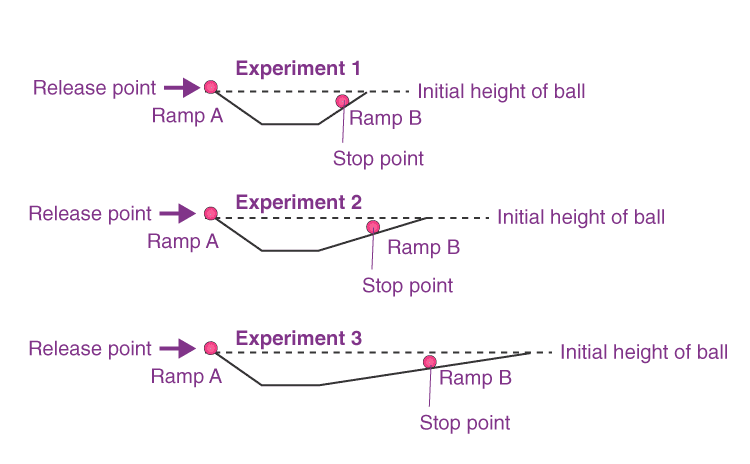Galileo used two inclined planes, as shown in the figure, and made the ball roll down the first plane and climb up the other. He concluded that, if the planes are smooth, the final height achieved by the ball is nearly the same as the height through which it rolls from the first plane. In the second experiment, the slope of the second inclined plane was decreased and the ball was made to roll again. Here, the ball still reaches the same height, and in doing so, it travels a longer distance. According to the observation, when the slope of the second plane was decreased to zero, that is, the plane was made horizontal, the ball was supposed to travel an infinite distance, that is the motion never ceases.
Although due to the opposing friction of the plane, the object does come to rest after a finite distance but under ideal conditions, when there is no friction, the ball would continue to move with constant velocity on the horizontal plane. With this conclusion, the statements of Aristotle were proved wrong. He concluded that it was incorrect to assume that a net force was needed to keep a body in uniform motion and the state of rest and state of uniform motion as equivalent.

What are the 3 Types of Inertia?

Following are the three types of inertia:

 Inertia of Rest Inertia of Direction Inertia of Motion When the resistance is offered by the body to continue in the state of rest unless an external force act on it. When the resistance is offered by the body to continue the motion in the same direction unless an external force act on it. When the resistance is offered by the body to continue to be in the uniform motion unless an external force act on it.

What are the Examples of Law of Inertia?

• Sudden start of the lift.
• The tendency of moving back when the stationary bus starts to move.

Newton's Laws of Motion - First Law

Introduction

Sir Isaac Newton published three laws in the 17th century. In this article, we are going to talk about Newton’s 1st law. This law is an introduction to motion of the object and the force acting on it. In other words, it deals with the motion of an object and its relation to force.

What is Newton’s First Law of Motion?

Newton’s first law states that

a body remains in the state of rest or uniform motion in a straight line unless and until an external force acts on it.

Putting Newton’s 1st law of motion in simple words, a body will not start moving until and unless an external force acts on it. Once it is set in motion, it will not stop or change its velocity until and unless some force acts upon it once more. The first law of motion is sometimes also known as the law of inertia.

There are two conditions on which the 1st law of motion is dependent:

• Objects at rest: When an object is at rest velocity (v= 0) and acceleration (a = 0) are zero. Therefore, the object continues to be at rest.
• Objects in motion: When an object is in motion, velocity is not equal to zero (v ≠ 0) while acceleration (a = 0) is equal to zero. Therefore, the object will continue to be in motion with constant velocity and in the same direction.

What is an External Force?

An external force is defined as the change in the mechanical energy that is either the kinetic energy or the potential energy in an object. These forces are caused by external agents. Examples of external forces are friction, normal force and air resistance.

Let us Understand First Law of Motion by an Example

Let us take a block on a smooth surface. By smooth, we mean that there is no friction acting on the surface. The block is at rest, that is, it is not moving. Now, let us examine the forces acting on the block. The only forces acting on the block are the force of gravity and the normal reaction by the surface. There is no force acting on it in the horizontal direction. Since the forces in the vertical direction are equal to each other in magnitude, they cancel each other out, and hence there is no external force on the block. Since this block is at rest, we can say that it confirms Newton’s first law of Motion.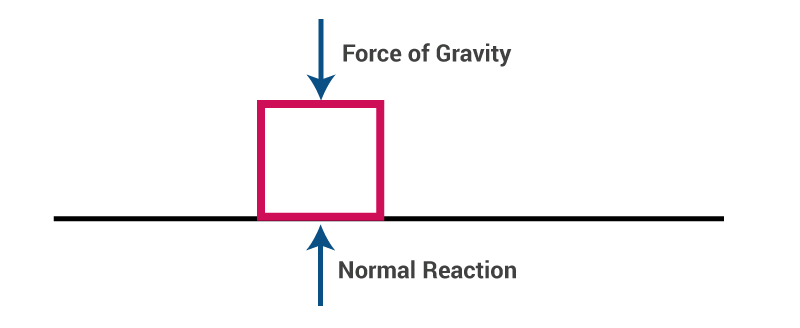Now, if we apply a constant force F on the block in a horizontal direction, it will start moving with some constant acceleration, in the direction of the applied force.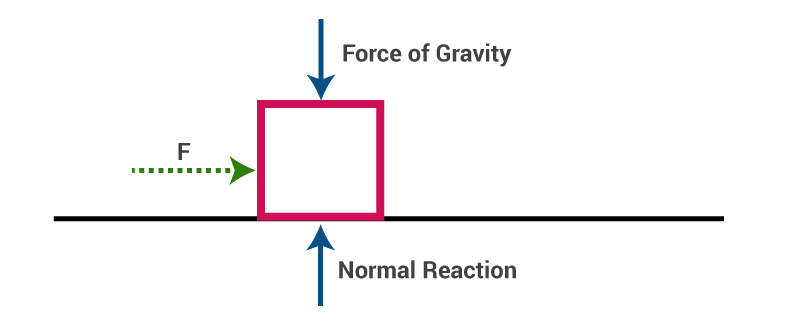Thus, the first law of motion is confirmed again.

Note: Newton’s laws are valid only in inertial frames of reference.

Newton’s First Law of Motion Examples in Daily Life

Wearing a seat belt in a car while driving is an example of Newton’s 1st law of motion. If an accident occurs, or if brakes are applied to the car suddenly, the body will tend to continue its inertia and move forward, probably proving fatal. To prevent such accidents seat belts are used which stops your body from moving forward in inertia avoiding danger.

Frequently Asked Questions – FAQs

What are the major concepts involved in the topic?
The major concepts involve Frame of reference, Newton’s First law of motion (Law of Inertia), Newton’s Second law of motion, Newton’s Third law of motion (For every action there is an equal and opposite reaction) and constraint equations.

What are the characteristics of normal force?
It is a force that always acts perpendicular to the surface of contact.

What do we understand by a free body diagram?
A free body diagram represents all the forces acting on the body. For example,So, the FBD for sphere B will be as shown below,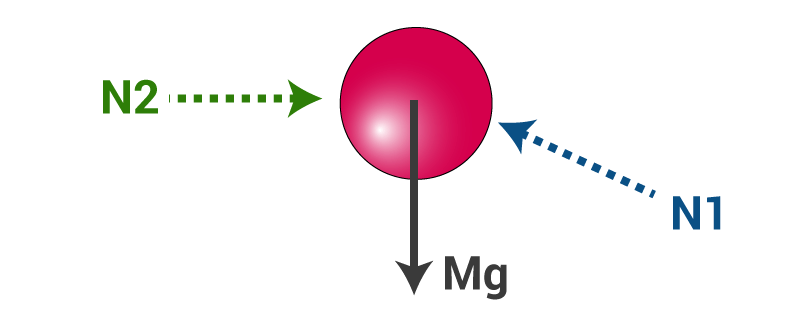What are the steps to solve a problem on Newton’s laws of motion?
Let us take the following example,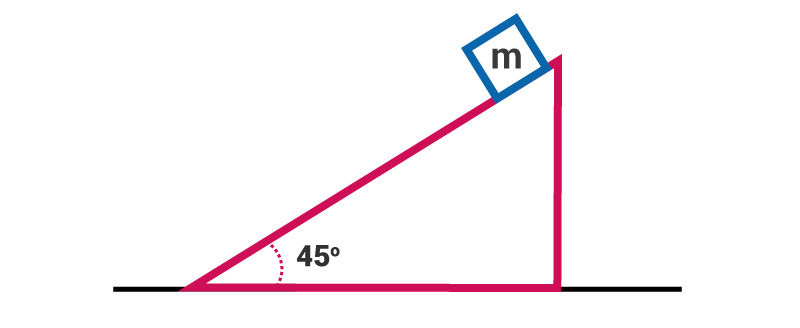The wedge is fixed, and we need to find the acceleration of the block of mass m along the incline.
Step 1: Draw the F.B.D of the block,Step 2: Writing the force equation taking our own axis, in this case, we take two axes one along the plane and the other perpendicular to it.
Fincline = mg sin45
VFNormal = N – mg cos45
Step3: Calculating acceleration using the second law of motion mg sin45 = ma

• a  = g sin45

What is the constraint equation?

When the motion of one body is governed by another or in other words when the motion of two or more bodies is interlinked. The equation governing such motion is known as the constraint equation. For example: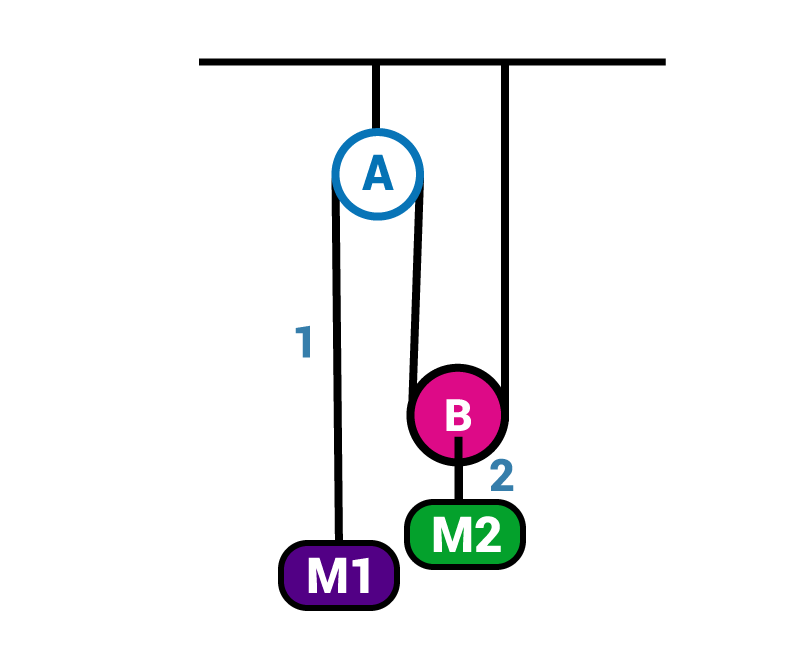If we draw the FBD and write equations we will get,
M1.g – T = M1.a1
2T – M2.g = M2.a2
We can see there are two equations and three unknowns: a1, a2 and T. So, we need one more equation and that will be the constraint equation.

The document Law of Inertia & First Law of Motion Notes | Study Physics Class 11 - NEET is a part of the NEET Course Physics Class 11.
All you need of NEET at this link: NEET

## Physics Class 11

126 videos|449 docs|210 tests
 Use Code STAYHOME200 and get INR 200 additional OFF

## Physics Class 11

126 videos|449 docs|210 tests

### How to Prepare for NEET

Read our guide to prepare for NEET which is created by Toppers & the best Teachers

Track your progress, build streaks, highlight & save important lessons and more!

,

,

,

,

,

,

,

,

,

,

,

,

,

,

,

,

,

,

,

,

,

;